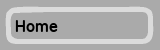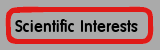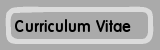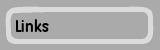Scientific Interests

Amplitude-exponent relations for 3D O(n) spin models

Making use of the conformal invariance of systems of statistical mechanics at a continuous phase transition, a rather complete understanding of two-dimensional critical phenomena is possible . Among the most prominent predictions of the resulting conformal field theory for critical systems in two dimensions are finite-size scaling laws for semi-finite geometries including the exact scaling amplitudes, in particular the scaling of correlation lengths on cylinders of finite circumference L . Although the bulk of exact results from conformal field theory is not available for three-dimensional systems, a similar scaling relation has been numerically found probable for the Ising model on the 3D geometry S1 x S1 x R with a special choice of boundary conditions .

Using high-precision Monte Carlo simulations and an elaborate set of data analysis tools, we examine the finite-size scaling behavior of correlation lengths of the more general O(n) spin models on this 3D geometry. We find that, for the Ising, XY and generalized Heisenberg models, a scaling relation of the type valid in 2D can be retained, when using antiperiodic instead of periodic boundary conditions [4,5]. For the case of periodic boundary conditions, a generalized scaling law is conjectured . A striking mismatch between the n -> oo extrapolation of the simulation results and analytical calculations is traced back to a breakdown of the identification of this limit with the spherical model . For another, conformally flat 3D geometry of the type S2 x R the relations valid in 2D can be extended to 3D by analytical means . Earlier attempts of a numerical reproduction of this result failed due to problems with the discretization of the sphere S2 . We use slightly irregular lattices for this discretization and find the generalized scaling relation valid for this geometry to high precision .

Dynamical quadrangulations and quantum gravity

Dynamical triangulations provide a discrete, non-perturbative approach to quantum gravity alternative to the various further directions pursued, including string theory, loop quantum gravity, non-commutative geometry or causal sets . Starting from a path-integral approach, the integral over metrics is being performed as a discrete sum over simplicial complexes of a given topology [11,12,13]. The Euclidean case in two dimensions can be solved exactly as a combinatorial problem by virtue of the powerful methods of matrix integrals and generating functions [14,15]. Additionally, matrix models for the coupling of certain matter variables such as Ising-, Potts or O(n) models to the triangulations can be formulated and partially solved . For the general case, the dressing of the weights of conformal matter of central charge c < 1 coupled to the Euclidean dynamical triangulations model in two dimensions is conjectured by the KPZ/DDK formula from Liouville theory [17,18,19], the predictions of which coincide with all known exact results.

We consider the coupling of the six-vertex F model to planar, "fat" \phi^4 Feynman diagrams corresponding to dynamical "quadrangulations". On regular lattices, vertex models exhibit rich phase diagrams  and the F model has a critical line of central charge c=1, i.e., directly at the "c=1 barrier'' where the KPZ/DDK description of the coupling of matter variables to the dynamical triangulations model breaks down. A matrix model for this problem can be formulated; its analytical treatment, however, does not reveal the detailed, finite-size behavior of most matter related and some graph related properties [21,22]. We thus analyze the coupled system with a set of Monte Carlo simulations of fluctuating graphs and coupled matter variables. While numerical simulations of dynamical triangulations have been extensively applied before, for the case of quadrangulations a set of update moves had to be devised first, where a suitable generalization of the "Pachner moves"  has to be augmented with additional "two-link flips" along self-energy subgraphs to ensure ergodicity . To alleviate the severe critical slowing down of the dynamics resulting from the fractal structure of the graphs, a non-local, cluster-type update in the form of the "baby-universe surgery" method is generalized to the quartic case [25,26]. From the regular-lattice system as well as the matrix model treatment, the system is expected to exhibit a Kosterlitz-Thouless phase transition to an anti-ferroelectrically ordered phase [20,21,22]. An order parameter for this transition on a random lattice can only be defined indirectly through a duality transformation of the model . The scaling analysis is found to be hampered by the combined effect of a comparable smallness of the effective linear extent of the graphs resulting from their large fractal dimension and the logarithmic corrections expected from a theory with central charge c=1. Nevertheless, a careful analysis of the results guided by a newly performed detailed analysis of the scaling behavior of the square-lattice model  confirms the predictions of the KPZ/DDK formula [17,18,19] with respect to the critical exponents related to the staggered polarization of the model. Concerning the location of the transition point and the order of the transition, agreement can be achieved with the matrix model results [21,22]. Additionally, various aspects of the back-reaction of the matter variables unto geometric properties of the graphs are investigated, including the co-ordination number distribution, the string susceptibility exponent and the internal Hausdorff dimension dh of the coupled system . With respect to the latter, we find dh = 4 as for the pure gravity model, in contradiction with two different analytical conjectures for the change of dh with the central charge c [28,29].

In an independent investigation, we consider the fractal properties of geometrical and Fortuin-Kasteleyn clusters of q = 2, 3 Potts models on dynamical triangulations and quadrangulations . On regular lattices, there exists an intimate relation between the critical properties of the pure Potts model and the tricritical point of the (annealed) diluted Potts model and the geometrical and Fortuin-Kasteleyn clusters of the pure model . Both sets of critical exponents are found to be related by a duality transformation preserving the central charge. We propose that this relation can be lifted to the level of fluctuating graphs by means of the KPZ/DDK formula and perform simulations and a finite-size scaling analysis of the Potts models on dynamical lattices to substantiate this claim .

Quenched disorder from random graphs

The Harris criterion judges the relevance of uncorrelated, quenched disorder for altering the universal properties of systems of statistical mechanics close to a continuous phase transition . In this case, a change of universality class is expected for models with a positive specific heat exponent α. For the physically more realistic case of spatially correlated disorder degrees of freedom, Harris' scaling argument can be generalized, yielding a shifted relevance threshold -oo < αc < 1 known as Luck criterion [33,34]. The value of αc depends on the quality and strength of the spatial disorder correlations as expressed in a so-called wandering exponent. We consider the effect of a different, topologically defined type of disorder on the universal behavior of coupled spin models, namely the result of connectivity disorder produced by placing spin models on random graphs. As it turns out, the Harris-Luck argument can be generalized to this situation, leading to a criterion again involving a suitably defined wandering exponent of the underlying random graph ensemble. Using a carefully tailored series of finite-size scaling analyses, we precisely determine the wandering exponents of the two-dimensional ensembles of Poissonian Voronoï-Delaunay random lattices as well as the quantum gravity graphs of the dynamical triangulations model, thus arriving at explicit predictions for the relevance threshold αc for these lattices .

According to these results, for the case of the Poissonian Voronoï-Delaunay random graphs the Harris criterion should stay in effect, whereas for the dynamical triangulations the threshold is shifted to the remarkably small value αc = -2. The latter result is in perfect agreement with Monte Carlo simulations of the q-states Potts model  as well as an available exact solution for the case of the percolation limit q -> 1 . For the Poissonian Voronoï-Delaunay triangulations, while the Ising case q = 2 with α = 0 is marginal and a change of universal properties cannot normally be expected, the q = 3 Potts model, exhibiting α = 1/3, should be shifted to a new universality class. Following up on a first exploratory study of this problem for small graphs , we perform high-precision cluster-update Monte Carlo simulations for rather large lattices of up to triangles to investigate this model. Astonishingly, however, the (exactly known) critical exponents of the square-lattice q = 3 Potts model are reproduced to high precision by our random-graph simulations [39,40]. To clarify this situation, a generalized model introducing a distance dependence of the interactions is currently under investigation [38,41].

Development and analysis of new Monte Carlo techniques

The multi-histogram method  is widely applied in Monte Carlo simulations near phase transition points. It allows for the combination of data from simulations at different points of the coupling parameter space for the evaluation of thermal averages as continuous functions of the coupling parameters. Generalizing the original scheme to the random-cluster representation of the q-state Potts model and making use of an exactly known property of the density of states in this formulation, we show how results for non-integer q values can be found from Monte Carlo simulations . We analyze the reasons for the failure of a similar previously proposed technique  and suggest a new method to circumvent the super-critical slowing down of the Potts model with large q values. In contrast to simulations in the usual energy/magnetization language, the use of cluster estimators enables a consistent definition of magnetization and magnetic susceptibility in the broken and unbroken phases even for finite-size lattices.

Finite-size scaling techniques enable a precise determination of transition temperatures and critical exponents. Often, different estimates for the same quantities are available. It is common practice to obtain final estimates from plain or variance-weighted means of the single estimates. A detailed analysis of the cross-correlations between these different estimates based on resampling techniques such as the jackknife method , shows that this approach leads to a systematic under-estimation of statistical errors and additionally introduces a bias . Instead, the weighting should be done taking the full covariance matrix into account.

Zero-temperature properties of spin-glass models with continuous symmetry

The problem of clarifying the phase structure and the static and dynamical properties of spin glass models has attracted an enormous amount of analytical and numerical work in the past decades [47,48]. Exploration of the landscape of ground states provides valuable insights in the low-temperature behavior of these systems . Due to their relative simplicity, Ising spin glass models such as the Edwards-Anderson (EA) Ising model have received much more attention than the continuous symmetry variants. As a consequence, the properties of the EA Ising model in two and three dimensions are now comparatively well understood, whereas for the in many cases more realistic continuous-symmetry cases even the fundamental question of the existence of a spin-glass phase transition is still a matter of recent debate .

Finding ground-states of spin glassy system is an inherently difficult problem known to be NP-hard in general . Thus, for the treatment of reasonably sized systems, approximation schemes have to be devised. For the analysis of (classical) O(n) vector spin glasses in two dimensions, we exploit the fact that the corresponding Ising model is one of the few cases exactly solvable in polynomial time through a mapping to a minimum-weight perfect matching problem  and apply the so-called "blossom algorithm" to Ising variables randomly embedded in the vector spins. Inserting this procedure into a specially tailored genetic algorithm using a cluster-exchange crossover operation allows to find numerically exact ground states for XY systems of up to 28 x 28 spins . In contrast to previous claims and widespread belief, we find the ground-state of the +/- J case to be unique up to a global O(n) transformation for finite systems. Comparing the disorder-averaged fluctuations of the ground-state energies between systems with periodic and anti-periodic boundary conditions allows for an estimation of the spin stiffness, for which contradicting predictions have been made from the replica-symmetry breaking and droplet pictures of the theory of spin glasses [47,48]. Utilization of various novel sets of boundary conditions and the so-called "aspect-ratio scaling" technique enable an independent and reliable determination of the spin and chiral stiffness exponents. The results, featuring different stiffness exponents for spin and chiral degrees-of-freedom, strongly support the occurrence of spin-chirality decoupling in this system, a much debated issue over which agreement could not be reached in the scientific community for the past 25 years .

Considering the intrinsically quantum nature of the physical systems modeled by the Edwards-Anderson spin glass, low-temperature quantum excitations should be taken into account for a proper description. This is done via a Holstein-Primakoff spin-wave approximation, modeling quantum excitations around the (classical) ground states found by the techniques outlined above. These calculations yield information about quantum corrections to the magnetization and susceptibilities, about the participation ratios and spin-wave velocities etc. .

References

1
J. L. Cardy, in Phase Transitions and Critical Phenomena, edited by C. Domb and J. L. Lebowitz (Academic Press, London, 1987), Vol. 11, p. 55.

2
J. L. Cardy, J. Phys. A 17, L385 (1984).

3
M. Henkel, J. Phys. A 20, L769 (1987).

4
M. Weigel and W. Janke, Ann. Phys. (Leipzig) 7, 575 (1998).

5
M. Weigel and W. Janke, Phys. Rev. Lett. 82, 2318 (1999).

6
M. Weigel and W. Janke, Phys. Rev. B 62, 6343 (2000).

7
J. L. Cardy, J. Phys. A 18, L757 (1985).

8
F. C. Alcaraz and H. J. Herrmann, J. Phys. A 20, 5735 (1987).

9
M. Weigel and W. Janke, Europhys. Lett. 51, 578 (2000).

10
L. Smolin, preprint hep-th/0303185.

11
V. A. Kazakov, I. Kostov, and A. A. Migdal, Phys. Lett. B 157, 295 (1985).

12
F. David, Nucl. Phys. B 257, 45 (1985).

13
J. Ambjørn, B. Durhuus, and J. Fröhlich, Nucl. Phys. B 257, 433 (1985).

14
E. Brézin, C. Itzykson, G. Parisi, and J.-B. Zuber, Commun. Math. Phys. 59, 35 (1978).

15
W. T. Tutte, Can. J. Math. 14, 21 (1962).

16
J. Ambjørn, B. Durhuus, and T. Jonsson, Quantum Geometry -- A Statistical Field Theory Approach (Cambridge University Press, Cambridge, 1997).

17
V. G. Knizhnik, A. M. Polyakov, and A. B. Zamolodchikov, Mod. Phys. Lett. A 3, 819 (1988).

18
F. David, Mod. Phys. Lett. A 3, 1651 (1988).

19
J. Distler and H. Kawai, Nucl. Phys. B 321, 509 (1989).

20
R. J. Baxter, Exactly Solved Models in Statistical Mechanics (Academic Press, London, 1982).

21
P. Zinn-Justin, Europhys. Lett. 50, 15 (2000).

22
I. Kostov, Nucl. Phys. B 575, 513 (2000).

23
M. Gross and S. Varsted, Nucl. Phys. B 378, 367 (1992).

24
M. Weigel and W. Janke, Nucl. Phys. B (Proc. Suppl.) 106-107, 986 (2002).

25
M. Weigel, Ph.d. thesis, University of Leipzig, 2002.

26
M. Weigel and W. Janke, Nucl. Phys. B 719, 312 (2005).

27
M. Weigel and W. Janke, J. Phys. A 38, 7067 (2005).

28
Y. Watabiki, Prog. Theor. Phys. Suppl. 114, 1 (1993).

29
N. Ishibashi and H. Kawai, Phys. Lett. B 322, 67 (1994).

30
M. Weigel and W. Janke, Geometric and Stochastic Clusters of Gravitating Potts Models, Preprint cond-mat/0604411.

31
W. Janke and A. M. J. Schakel, Nucl. Phys. B 700, 385 (2004).

32
A. B. Harris, J. Phys. C 7, 1671 (1974).

33
J. M. Luck, Europhys. Lett. 24, 359 (1993).

34
A. Weinrib and B. I. Halperin, Phys. Rev. B 27, 413 (1983).

35
W. Janke and M. Weigel, Phys. Rev. B 69, 144208 (2004).

36
W. Janke and D. A. Johnston, Nucl. Phys. B 578, 681 (2000).

37
V. A. Kazakov, Mod. Phys. Lett. A 4, 1691 (1989).

38
F. W. S. Lima, U. M. S. Costa, M. P. Almeida, and J. S. Andrade, Eur. Phys. J. B 17, 111 (2000).

39
W. Janke and M. Weigel, Acta Phys. Polon. B 34, 4891 (2003).

40
W. Janke, D. A. Johnston and M. Weigel, Condens. Matter Phys. 9, 263 (2006).

41
G. Kaehler, Diploma thesis, University of Leipzig, 2004.

42
A. M. Ferrenberg and R. H. Swendsen, Phys. Rev. Lett. 63, 1195 (1989).

43
M. Weigel, W. Janke, and C.-K. Hu, Phys. Rev. E 65, 036109 (2002).

44
C.-K. Hu, Phys. Rev. B 46, 6592 (1992).

45
B. Efron, The Jackknife, the Bootstrap and Other Resampling Plans (Society for Industrial and Applied Mathematics [SIAM], Philadelphia, 1982).

46
M. Weigel and W. Janke, in preparation.

47
K. Binder and A. P. Young, Rev. Mod. Phys. 58, 801 (1986).

48
N. Kawashima and H. Rieger, in Frustrated Spin Systems, edited by H. T. Diep (World Scientific, Singapore, 2005), Chap. 6.

49
A. K. Hartmann and H. Rieger, Optimization Algorithms in Physics (Wiley, Berlin, 2002).

50
L. W. Lee and A. P. Young, Phys. Rev. Lett. 90, 227203 (2003).

51
I. Bieche, R. Maynard, R. Rammal, and J. P. Uhry, J. Phys. A 13, 2553 (1980).

52
M. Weigel and M. J. P. Gingras, Phys. Rev. Lett. 96, 097206 (2006).

53
M. Weigel and M. J. P. Gingras, in preparation.

 Webmaster: Martin Weigel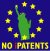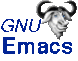Timestamp: Thu Jun 15 14:51:01 2006